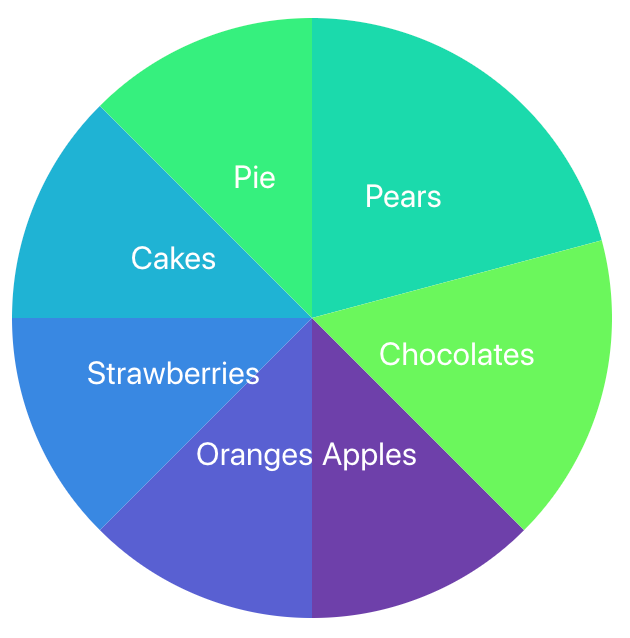# Implementing a Pie Chart using React and D3.js

Today, we will be looking into how to create a Pie Chart using React and D3.js

### Creating a new component

The basic structure of our `<PieChart />` component will look like this:

``````import React, { useEffect } from 'react';
import * as d3 from 'd3';

function PieChart(props) {
const {
data,
} = props;
const margin = {
top: 50, right: 50, bottom: 50, left: 50,
};
const width = 2 * outerRadius + margin.left + margin.right;
const height = 2 * outerRadius + margin.top + margin.bottom;
useEffect(() => {
drawChart();
}, [data]);

function drawChart() {
// draw the chart here
}

return <div id="pie-container" />;
}

export default PieChart;``````

Let us take a look at the different props it will be using — `margin` and `outerRadius` are used to calculate the `width` and `height` of our pie. By having `innerRadius` greater than 0, we can very easily convert our pie chart into a donut chart as well 🍩 😄

Our component will render a `div` element which will be containing the Pie Chart.

`drawChart` function will be responsible for actually drawing our pie chart. It will be called every time the `data` prop changes.

#### Defining the data

`PieChart` will accept an array of objects for the `data` prop. This is how it will look like —

``const data = [{ label: 'Apples', value: 10 }, { label: 'Oranges', value: 20 }];``

### Drawing the Pie

Now, let’s populate the `drawChart` function. Everything below will go inside this function:

#### Creating a color scale

First, let us define what interpolations is —

Interpolation is the process of estimating unknown values that fall between known values.

``````const colorScale = d3
.scaleSequential()
.interpolator(d3.interpolateCool)
.domain([0, data.length]);``````

The d3-interpolate module provides a variety of interpolation methods for blending between two values. Values may be numbers, colors, strings, arrays, or even deeply-nested objects.

The scaleSequential method maps a continuous domain to a continuous range defined by an interpolator function. We are using the d3.interpolateCool color scale but you can use any other as well.d3.interpolateCoold3.interpolateWarm

In simple words, any value falling between `[0, data.length]` will be mapped to a value in the given color scale. We will be using these colors so that each sector of our pie has a different color.

#### Appending SVG

``````// Remove the old svg
d3.select('#pie-container')
.select('svg')
.remove();
// Create new svg
const svg = d3
.select('#pie-container')
.append('svg')
.attr('width', width)
.attr('height', height)
.append('g')
.attr('transform', `translate(\${width / 2}, \${height / 2})`);``````

We first clear the previous chart before drawing a new one (remember `drawChart` is called every time the `data` changes?)

We append an `svg` inside the `div` element we rendered. We also append `g` inside the `svg` to group our elements together to apply easy transformations to them. We translate this group of elements to the center of the `svg`

#### Defining the generators

``````const arcGenerator = d3
.arc()
const pieGenerator = d3
.pie()
.value((d) => d.value);
const arc = svg
.selectAll()
.data(pieGenerator(data))
.enter();``````

`d3.arc` doesn’t actually draw any arcs yet but it is used to create an `arcGenerator` which will be used to draw the sectors of the pie. Similarly, `d3.pie` doesn’t draw the pie but it used to compute the necessary angles to represent our data as a pie.

We then enter the svg group with the computed data. Now, we are ready to actually draw the pie.

``````// Append sectors
arc
.append('path')
.attr('d', arcGenerator)
.style('fill', (_, i) => colorScale(i))
.style('stroke', '#ffffff')
.style('stroke-width', 0);
// Append text labels
arc
.append('text')
.attr('text-anchor', 'middle')
.attr('alignment-baseline', 'middle')
.text((d) => d.data.label)
.style('fill', '#ffffff)
.attr('transform', (d) => {
const [x, y] = arcGenerator.centroid(d);
return `translate(\${x}, \${y})`;
});``````

We append a path for each sector. If you notice carefully, we have used the index of each data item to map to the color scale which is used as a fill color for the sector.

Next, we append text elements for each data item and position them using `arcGenerator.centroid` which returns the center of each sector. That’s it!

This is how your pie chart will look like ✨